##### ASVAB AFQT For Dummies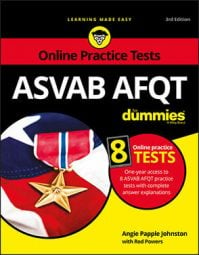Dividing decimals without a calculator can be a challenge, but it's necessary on the ASVAB AFQT. When you divide decimals, you have to use both subtraction and multiplication. You also need to be pretty good at rounding and estimating numbers. Don't worry, though. You can divide decimals in one of two ways: long division and conversion.

## Method 1: Dividing decimals using long division

To do long division with decimals, follow these steps:
1. If the divisor isn't a whole number, move the decimal point in the divisor all the way to the right (to make it a whole number), and move the decimal point in the dividend the same number of places to the right.
2. Position the decimal point in the result directly above the decimal point in the dividend.
3. Divide as usual.

If the divisor doesn't go into the dividend evenly, add zeros to the right of the last digit in the dividend and keep dividing until it comes out evenly or a repeating pattern shows up.

Try the following division problem: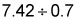Write the problem on your scratch paper in long-division form: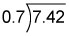Now move the decimal point one place to the right, which makes the divisor a whole number. Also move the decimal point in the dividend one place to the right: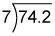Position the decimal point in the result directly above the decimal point in the dividend: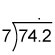Divide as usual. Seven goes into 70 ten times with 4 left over; then drop the 2 down: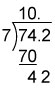Seven goes into 42 six times: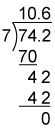Remember: when you're finished dividing decimals, you're finished. You don't have to move the decimal point around like you do after you've multiplied decimals.

## Method 2: Dividing decimals using conversion

The other way to divide decimals is to convert the decimals to fractions and then divide the fractions.

Try the problem again, this time using the conversion method.First, convert the decimals to fractions: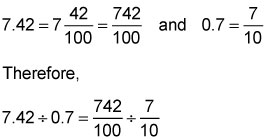Take the reciprocal of the divisor (flip the second fraction upside down) and then multiply: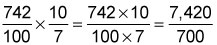The fraction 7,420/700 can be simplified to 10 3/5. Convert 3/5 to a decimal, and the answer is 10.6.